# A Note on Boxplots in R

As a statistical consultant I frequently use boxplots. They're a great way to quickly visualize the distribution of a continuous measure by some grouping variable. More often than not, however, the person I'm helping doesn't regularly use boxplots (if at all) and is not sure what to make of them.

Fortunately, boxplots are pretty easy to explain. The line in the middle of the box is the median. The box itself represents the middle 50% of the data. The box edges are the 25th and 75th percentiles. But what about the whiskers? That seems to be the part that trips people up.

Before we go further, let's make some boxplots in R:

set.seed(9)
y <- rnorm(n = 100, mean = rep(c(22:25),each=25),sd = rep(c(5,8),each=50))
x <- gl(n=4,k=25)
boxplot(y ~ x)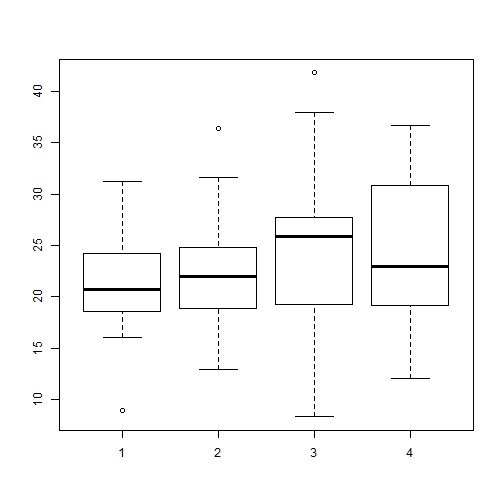Above I generate 100 random normal values, 25 each from four distributions: N(22,5), N(23,5), N(24,8) and N(25,8). Then I generate a 4-level grouping variable. Finally I make the boxplot.

The black lines in the “middle” of the boxes are the median values for each group. For group 1, that appears to be a shade above 20. The vertical size of the boxes are the interquartile range, or IQR. They measure the spread of the data, sort of like standard deviation. The tops and bottoms of the boxes are referred to as “hinges”. We see groups 1 and 2 have less variability than groups 3 and 4, which makes sense given the way we generated the data. The IQR for group 1 looks to be about 5 judging from the height of the box. Again this makes sense given group 1 data was randomly generated from a normal distribution with a standard deviation of 5.

And now we come to the “whiskers”, or the flattened arrows extending out of the box. What do they represent and how are they calculated? They represent the reasonable extremes of the data. That is, these are the minimum and maximum values that do not exceed a certain distance from the middle 50% of the data. What is that certain distance? By default in R, it's $$1.5 \times IQR$$. If no points exceed that distance, then the whiskers are simply the minimum and maximum values. That's the case in group 4. If there are points beyond that distance, the largest point that does not exceed that distance becomes the whisker. And the points beyond the distance are plotted as single points.

For groups 1 through 3, there appear to be single points beyond the whiskers. (I say “appear to be single” because technically we could have overplotting.) We might think of these as outliers, data points that are too big or too small compared to the rest of the data. Group 4 does not appear to have outliers.

When you create a boxplot in R, you can actually create an object that contains the plotted data. Just call the boxplot as you normally would and save to a variable.

bp <- boxplot(y ~ x, plot = F)
bp

## $stats ## [,1] [,2] [,3] [,4] ## [1,] 16.06564 12.90309 8.300651 12.05522 ## [2,] 18.53334 18.90152 19.281150 19.14307 ## [3,] 20.75958 21.98459 25.924704 23.00494 ## [4,] 24.18153 24.84778 27.721310 30.84133 ## [5,] 31.22629 31.65432 38.016463 36.66171 ## ##$n
##  25 25 25 25
##
## $conf ## [,1] [,2] [,3] [,4] ## [1,] 18.97475 20.10557 23.25761 19.30830 ## [2,] 22.54441 23.86361 28.59179 26.70159 ## ##$out
##   8.911472 36.409950 41.843672
##
## $group ##  1 2 3 ## ##$names
##  "1" "2" "3" "4"


We see the bp object is a list with 6 different elements. The first element, stats, contains the plotted points in each group. So looking at column 1, we see that the bottom and top whiskers are 16.0656374 and 31.226286, the 25th and 75th percentiles are 18.5333406 and 24.1815345, and the median is 20.759577.

The out element of the bp object contains the outliers while the group element contains the outliers' respective groups.

If you want to change how whiskers are determined, you can change the range argument in the boxplot function. The default setting is 1.5. Here we try it set to 1:

boxplot(y ~ x, range=1)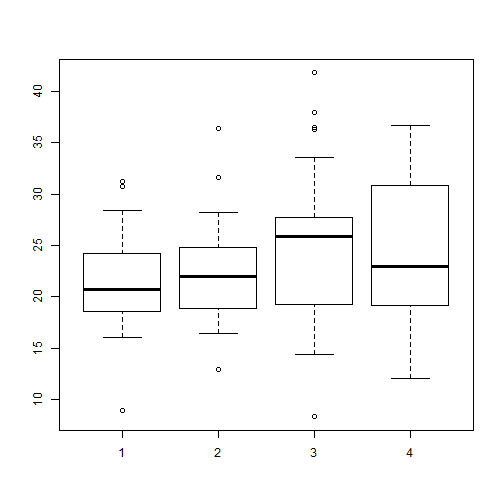Now we have more “outliers” for groups 1 – 3 but still none for group 4. Obviously the “outlier” classification is subjective. You'll also notice the size of the boxes didn't change. They will always capture the middle 50% of the data no matter the value of range. If we set range to 0, then the whiskers will extend to the minimum and maximum values for each group. In that case you get a plot of what is known as Tukey's Five-number summary: minimum, 25th percentile, median, 75th percentile and the maximum. In fact there's a function in R to calculate the Five-Number summary called fivenum. Here's how we can use it on our data:

tapply(y,x,fivenum)

## $1 ##  8.911472 18.533341 20.759577 24.181534 31.226286 ## ##$2
##  12.90309 18.90152 21.98459 24.84778 36.40995
##
## $3 ##  8.300651 19.281150 25.924704 27.721310 41.843672 ## ##$4
##  12.05522 19.14307 23.00494 30.84133 36.66171

boxplot(y ~ x, range=0)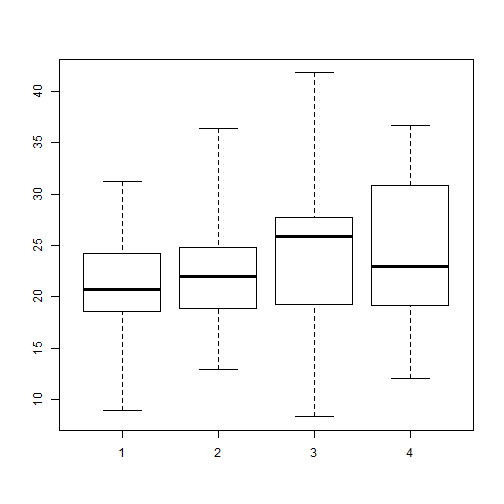A nice complement to boxplots are stripcharts. These are one-dimensional scatter plots of data. Here's out it works “out of the box”:

stripchart(y ~ x)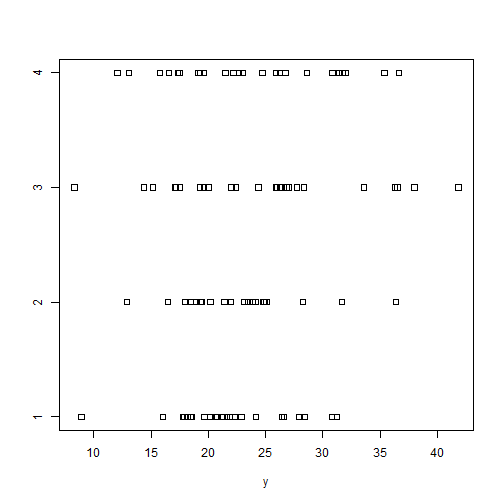I usually like to rotate stripcharts and use the familiar open circles with some “jitter”:

stripchart(y ~ x, vertical = TRUE, pch=1, method="jitter")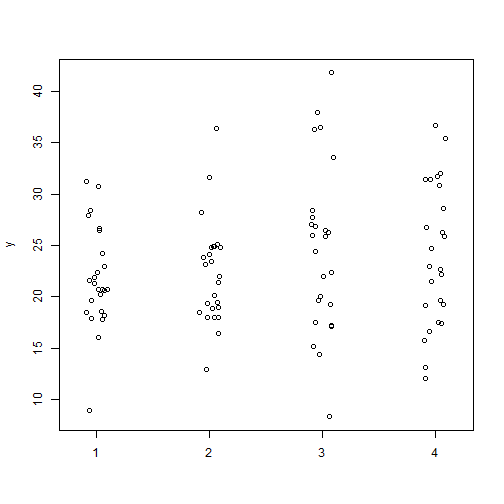Plotting stripcharts and boxplots side-by-side can be useful to visualize the spread and distribution of data. You can also calculate means and medians add them with the points function:

op <- par(mfrow=c(1,2))
means <- tapply(y,x,mean) # calculate means
medians <- tapply(y,x,median) # calculate medians
boxplot(y ~ x, ylab="y")
points(x = 1:4,y=means,pch=19) # add means
stripchart(y ~ x, vertical = TRUE, pch=1, method="jitter")
points(x = 1:4,y=means,pch=19, col="blue") # add means
points(x = 1:4,y=medians,pch=19, col="red") # add means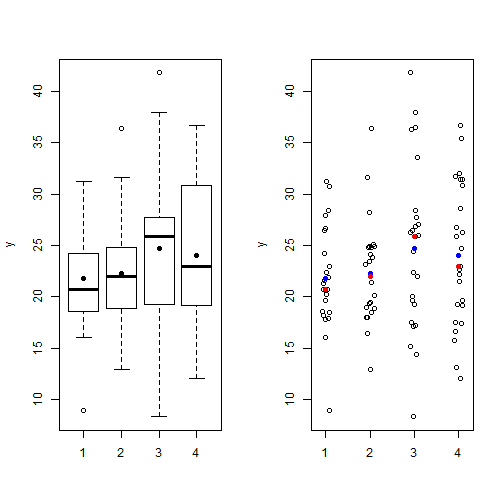par(op) # reset graphics parameters